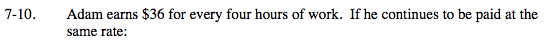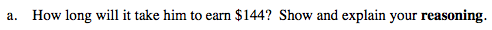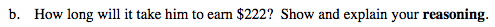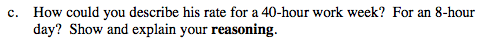### Home > MC2 > Chapter Ch7 > Lesson 7.1.1 > Problem7-10

7-10.$144 ÷$36 = 4 sets of 4 hours each

16 hours$222 ÷$36 = how many sets of 4 hours each?How many sets of 4 hours are there in 40 hours? 8 hours?
If Adam makes $36 for every 4 hours, how much does this total?$360 for a 40-hour week, \$72 for an 8-hour day.